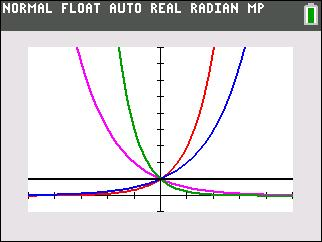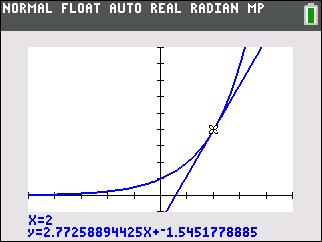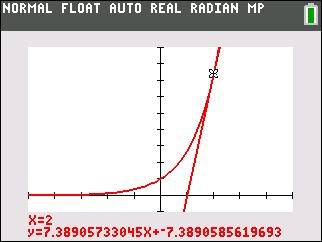### Mathematics lessons for IB® Diploma Programme

Applications and Interpretation | Numbers and Algebra

## Exponential Dice

### Objectives• Students will analyze data determined by a simulation involving tossing dice.
• Students will find and analyze exponential growth and decay functions.
• Students will model with mathematics.
• Students will try to make a connection with how to understand these topics in IB Mathematics courses and on their final assessments.

### Vocabulary

• exponential decay function
• half-life
• simulation
• exponential growth function
• doubling time

• This activity is done with the use of the TI-84 family as an aid to the problems, specifically using Lists and the Probability Simulation App.

## Key Steps## Step 1

In Problem 1, students investigate the how the value of b affects the shape of the graph y = bx.## Step 2

In Problem 2, students use a line tangent to the curve at a point to explore the relationship between the slope of the tangent line at that point and the value of the function at the same point.## Step 3

At the end of this activity, students will discover that there is exactly one value of b, Euler’s number, for which the slope of the tangent and value of the function are equal.

Students will also explore and model applications of exponential growth in given situations.